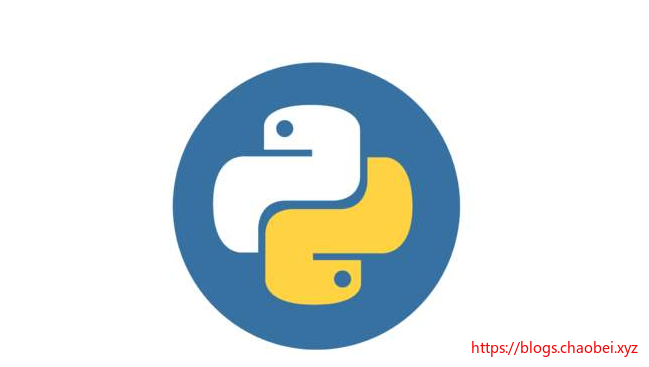# PYTHON 学习笔记2 流程控制工具以及函数定义

• if 条件判断
• for 循环
• while 循环

## if-elif-else 判断

``````
num = int(input("输入一个数字  :"))

if num > 10:
print("input > 10")
elif num >=5:
print("input >= 5")
else:
print("input < 5")

``````

``````输入一个数字  :7
input >= 5
``````

## while 循环

while 循环也算是一种常见的循环方式，这种循环一般要在循环体类结束循环，或者通过条件的方式让其结束。并不能无限循环下去。

``````
a,b = 0,1

while a < 100 :

print(a,end=',')

a,b = b,a+b

print("循环结束")

``````
• `a < 100` 作为条件体，若满足条件返回true ,则执行循环体

## while-else

while-else 则是在条件块不满足的时候，只会执行一次的代码块：

``````a,b = 0,1

while a < 100 :

print(a,end=',')

a,b = b,a+b
else:
print("条件不满足")

print("跳出循环")
``````

## break 与 continue

• break 则停止所有循环
• continue 则跳出本次循环
• 但不会在循环被 break 语句终止时被执行
``````a = 0
while a < 100 :

a=a+1

if a % 2 != 0 :
continue

print("偶数",a)
``````

``````
a = 0
while a < 100 :
a=a+1
if a == 33:
break
print(a)

``````

## for 循环

for in 一般用于List 列表的遍历。

``````>>> words = ['a','abc','abcd']
>>> for item in words :
print(item,len(item))

a 1
abc 3
abcd 4
``````
• item 用于表示当前项
• len() 函数用于计算字符串的长度

## for-else

``````for i in range(10):
print(i)

else:
print("循环结束")
``````

else 则是在循环结束后（不满足条件后）执行的代码块，

### range() 函数

range() 函数则是为了生成指定范围内的自然数，

``````range(5, 10)
5, 6, 7, 8, 9

# 这里的3 则表示步进
range(0, 10, 3)
0, 3, 6, 9

# 步进也可以是复数
range(-10, -100, -30)
-10, -40, -70
``````

## pass

pass 语句什么也不做。当语法上需要一个语句，但程序需要什么动作也不做时，可以使用它。

``````while True:
pass
print('a')

print("end")
``````

## 定义函数 def

``````def fib(n):

result = []

a,b = 0,1

while a<n :

result.append(a)

a,b = b,a+b

return result

print("fib start")

print(fib(100))
``````

• 若函数内部不带有 `return` 则会返回一个 `None`
``````def demo():
print("no return")

print(demo())
----------------------
no return
None

``````

### 默认值定义法

``````def other(a,b=5):

for i in range(b):
print(i,"-",a)

other(10)
-------------------------
0 - 10
1 - 10
2 - 10
3 - 10
4 - 10

``````

### 关键词参数

``````def other(a,b=5):

for i in range(b):
print(i,"-",a)

other(a=111,b=3)
--------------------
0 - 111
1 - 111
2 - 111
``````

### 特殊函数

``````
def f(pos1, pos2, /, pos_or_kwd, *, kwd1, kwd2):
-----------    ----------     ----------
|             |                  |
|        Positional or keyword   |
|                                - Keyword only
-- Positional only

``````

`/` 后方的参数既可以用位置参数 或者是 关键词参数
`/` 前方的参数必须是位置参数
`*` 后方的参数只能使用关键字参数

``````
# 基本形式
def standard_arg(a):
print(a)

# 仅限位置
def pos_only_arg(a,/):
print(a)
# 仅限关键字参数
def kwd_only_arg(*,a):
print(a)

def combined_example(pos_only, /, standard, *, kwd_only):
print(pos_only, standard, kwd_only)

``````
• 基本形式：可以传递任意形式
``````standard_arg('hello')
standard_arg(a ='hello')
-----------------------
hello
hello
``````
• 仅限位置参数
``````pos_only_arg('world')
#以下形式会报错
#pos_only_arg(a='world')
``````
• 仅限关键字参数
``````kwd_only_arg(a='python')
# 以下形式会报错，不可使用
#kwd_only_arg('python')
``````
• 双重形式
``````combined_example('hello',standard='world',kwd_only='python')

combined_example('hello','world',kwd_only='python')
----------------------

hello world python
hello world python

``````

## Lambda 表达式

Lambda函数可以在需要函数对象的任何地方使用。它们在语法上限于单个表达式。从语义上来说，它们只是正常函数定义的语法糖。与嵌套函数定义一样，lambda函数可以引用所包含域的变量:

``````def create_fun(n):

return lambda x:x+n

f = create_fun(50)

print(f(1))
------------------
51
``````

`f = create_fun(50)` 已经创建了一个匿名函数，并且赋值给 f，现在 f 就是一个匿名函数，可以传参，通过`f(1)` 调用匿名函数后，1其实就是匿名函数的参数 x, 做一个相加后，则返回的就是 51